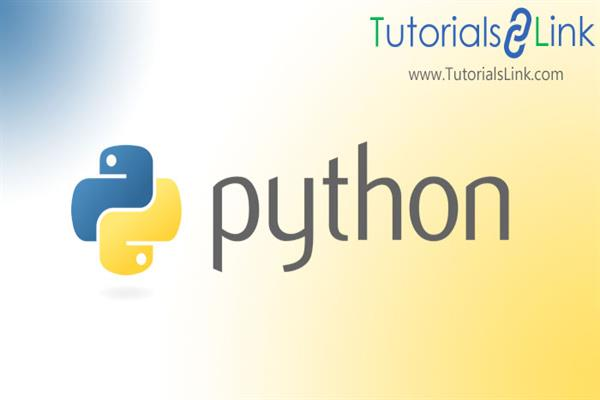Loading, please wait...# How to Broadcast with NumPy Array | python Tutorial

we can perform the arithmetic operation in two ways.

1. using scalars

2. between two or more arrays.

The arithmetic operation in NumPy is the element by element processes but the size and the shape arrays of arrays must be the same.

Then what about the arrays of different size and shape. Can we perform an arithmetic operation on NumPy arrays with different sizes and shapes? using the above approach we

Can't perform an arithmetic operation on NumPy arrays with different sizes and shapes.

Let’s look at an example code for better understanding.

``````import numpy as np

arr =np.array([1,2,3,4])

newarr=arr+2

print(newarr)

#output: [3 4 5 6]``````

Code Explanation:

We successfully perform an arithmetic operation on numpy arrays using scalar, but how is that possible? Because 2 is a single element or a constant value and arr is an array, i.e a group of elements. So how is this possible to perform an arithmetic operation on arrays using a scalar value..? This is possible because of broadcasting. So broadcasting is a technique in NumPy that allows us to perform an arithmetic operation on arrays of different sizes and shapes. So that means we can perform an arithmetic operation on arrays of different sizes and shapes using broadcasting techniques.

Broadcasting stretches the value or the arrays to the required shape and then performs an arithmetic operation. but NumPy doesn’t perform this with stretching and replicating. It directly performs this. To perform broadcasting we must follow some rules and those rules are known as Broadcasting rules. So let’s discuss those rules.

Rule1: If the two arrays differ in their number of dimensions, the shape of the one with fewer dimensions is padded with ones on its leading side(left side).

Rule2: If the shape of two arrays doesn’t match in any dimension, the array with shape equal to 1 in that dimension is stretched to match the other shape.

Rule 3: If in any dimension that sizes disagree and neither equal to 1, an error is raised.

``````

import numpy as np

arr1 =np.array([[1,2],[3,4],[5,6]])

arr2 =np.array([1,2,3])

print(arr1.shape)

#output: (3,2)

print(arr2.shape)

#output:(3,)

#print(arr1+arr2)

#output:ValueError: operands could not be broadcast together with shapes (3,2) (3,)

``````

Explanation 1:

Here array arr1 is of dimension (3, 2) but arr2 is of dimension (3,)

So on applying Rule 1, the dimension of arr2 becomes (1,3).

Now according to rule 2, we will compare the column of arr1 with the column of arr2.

It doesn’t match so we will check another condition whether the size of one of the dimensions is 1? No. hence, it will not check other sizes, and raise a ValueError.

Example 2: broadcasting 1-D numpy array:

``````import numpy as np

arr1 =np.array([[1,2],[3,4],[5,6]])

arr3=np.array([8,9])

print(arr1.shape)

#output: (3,2)

print(arr3.shape)

#output:(2,1)

print(arr1+arr3)

#output:

[[ 9 11]

[11 13]

[13 15]]``````

Explanation2: here array arr1 is of dimension (3,2) but arr3 is of dimension (2,).

So on applying Rule 1, the dimension of arr3 becomes (1,2).

Now we will compare the column of arr1 with the column of arr3 and here it matches

we will check other condition the size of the rows of arr1 and arr3, It doesn’t match so we will check another condition whether the size of one of the dimension is 1, the answer is yes

so, broadcasting will happen and return the array with a higher dimension.

``````import numpy as np

arr1 =np.array([,,])

arr3=np.array([8,9,10])

print(arr1.shape)

#output: (1,3)

print(arr3.shape)

#output:(3,)

print(arr1+arr3)

#output:

[[ 9 10 11]

[11 12 13]

[13 14 15]]

``````

Explanation:

Here, array arr1 is of dimension (3,1) but arr3 is of dimension (3,).

So on applying Rule 1, the dimension of arr3 becomes (1,3).

Now we will compare a column of arr1 with the column of arr3 and here it doesn’t match so we will check another condition whether the size of one of the dimensions is 1, the answer is yes both have one dimension as 1.

so, broadcasting will happen and return the array with a higher dimension.

Thanks for reading the article, I just hope you like the content. don’t forget to comment and share. Stay tuned with us for more useful content.

Till now,

Happy learning